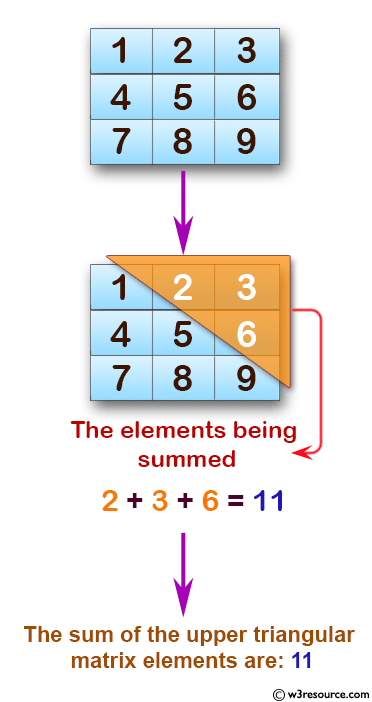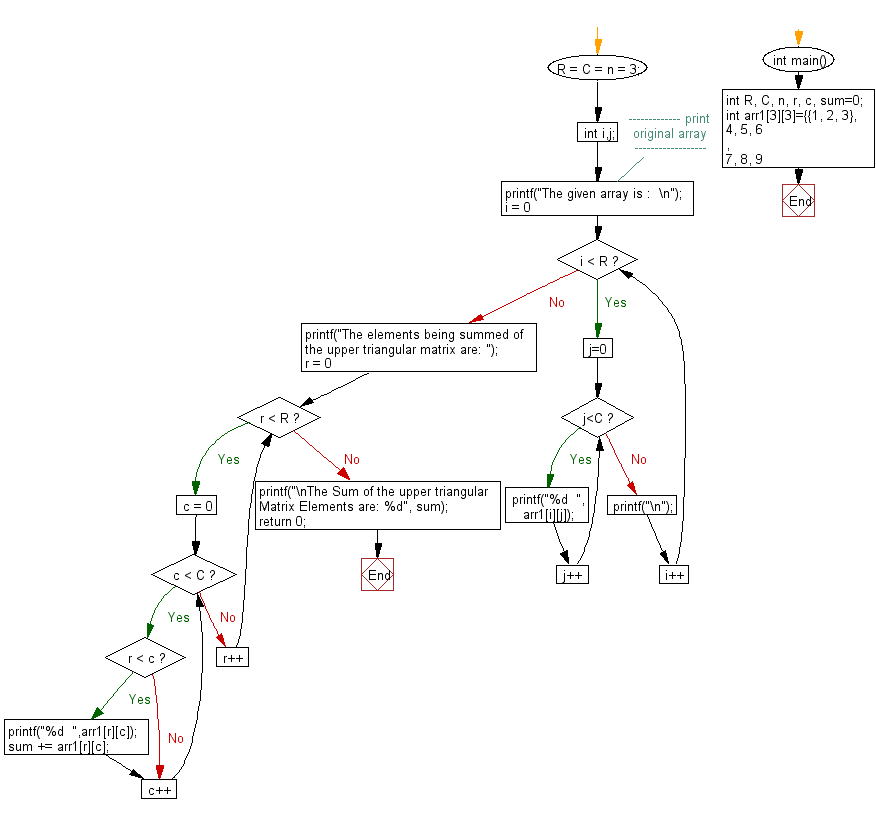﻿ C : Find the sum of upper triangular elements of a matrix

# C Exercises: Find the sum of upper triangular elements of a matrix

## C Array: Exercise-74 with Solution

Write a program in C to find the sum of the upper triangular elements of a matrix.

Visual Presentation:Sample Solution:

C Code:

``````#include <stdio.h>

int main() {
int R, C, n, r, c, sum = 0;
int arr1 = {{1, 2, 3},
{4, 5, 6},
{7, 8, 9}};
R = C = n = 3;
int i, j;

// Print original array
printf("The given array is :  \n");
for (i = 0; i < R; i++) {
for (j = 0; j < C; j++) {
printf("%d  ", arr1[i][j]);
}
printf("\n");
}

printf("The elements being summed of the upper triangular matrix are: ");
for (r = 0; r < R; r++) {
for (c = 0; c < C; c++) {
if (r < c) {
printf("%d  ", arr1[r][c]);
sum += arr1[r][c]; // Add the elements of the upper triangular matrix
}
}
}
printf("\nThe Sum of the upper triangular Matrix Elements are: %d", sum);
return 0;
}
```
```

Sample Output:

```The given array is :
1  2  3
4  5  6
7  8  9
The elements being summed of the upper triangular matrix are: 2  3  6
The Sum of the upper triangular Matrix Elements are: 11
```

Flowchart:C Programming Code Editor:

What is the difficulty level of this exercise?

Test your Programming skills with w3resource's quiz.

﻿